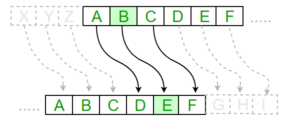Substitution Cipher

• Difficulty Level : Medium
• Last Updated : 29 Sep, 2021

Hiding some data is known as encryption. When plain text is encrypted it becomes unreadable and is known as ciphertext. In a Substitution cipher, any character of plain text from the given fixed set of characters is substituted by some other character from the same set depending on a key. For example with a shift of 1, A would be replaced by B, B would become C, and so on.

Note: Special case of Substitution cipher is known as Caesar cipher where the key is taken as 3.

Mathematical representation

The encryption can be represented using modular arithmetic by first transforming the letters into numbers, according to the scheme, A = 0, B = 1,…, Z = 25. Encryption of a letter by a shift n can be described mathematically as.(Encryption Phase with shift n)(Decryption Phase with shift n)Examples:

Plain Text: I am studying Data Encryption
Key: 4
Output: M eq wxyhCmrk Hexe IrgvCtxmsr

Plain Text: ABCDEFGHIJKLMNOPQRSTUVWXYZ
Key: 4
Output: EFGHIJKLMNOPQRSTUVWXYZabcd

Algorithm for Substitution Cipher:

Input:

• A String of both lower and upper case letters, called PlainText.
• An Integer denoting the required key.

Procedure:

• Create a list of all the characters.
• Create a dictionary to store the substitution for all characters.
• For each character, transform the given character as per the rule, depending on whether we’re encrypting or decrypting the text.
• Print the new string generated.

Below is the implementation.

Python3

 # Python program to demonstrate# Substitution Cipher  import string  # A list containing all charactersall_letters= string.ascii_letters       """create a dictionary to store the substitutionfor the given alphabet in the plain textbased on the key"""       dict1 = {}key = 4  for i in range(len(all_letters)):    dict1[all_letters[i]] = all_letters[(i+key)%len(all_letters)]    plain_txt= "I am studying Data Encryption"cipher_txt=[]  # loop to generate ciphertext  for char in plain_txt:    if char in all_letters:        temp = dict1[char]        cipher_txt.append(temp)    else:        temp =char        cipher_txt.append(temp)         cipher_txt= "".join(cipher_txt)print("Cipher Text is: ",cipher_txt)       """create a dictionary to store the substitutionfor the given alphabet in the ciphertext based on the key"""       dict2 = {}    for i in range(len(all_letters)):    dict2[all_letters[i]] = all_letters[(i-key)%(len(all_letters))]      # loop to recover plain textdecrypt_txt = [] for char in cipher_txt:    if char in all_letters:        temp = dict2[char]        decrypt_txt.append(temp)    else:        temp = char        decrypt_txt.append(temp)         decrypt_txt = "".join(decrypt_txt)print("Recovered plain text :", decrypt_txt)

Output:

Cipher Text is:  M eq wxyhCmrk Hexe IrgvCtxmsr
Recovered plain text : I am studying Data Encryption

Attention geek! Strengthen your foundations with the Python Programming Foundation Course and learn the basics.

To begin with, your interview preparations Enhance your Data Structures concepts with the Python DS Course. And to begin with your Machine Learning Journey, join the Machine Learning - Basic Level Course

My Personal Notes arrow_drop_up